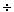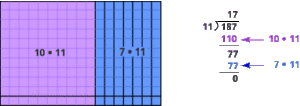# Meanings and Models for Operations Part B: Area Models for Multiplication and Division (45 minutes)

In This Part: Multiplication with Manipulatives
Models and manipulative materials can help make multiplication and division with integers more tangible. They provide a visual representation of the algorithms we use to perform these operations and help us see how the algorithms relate to what is actually happening with the manipulatives.

In this and the following area model examples, we will use three sizes of manipulatives: large 10 • 10 squares (we’ll call them flats); 1 • 10 rectangles (we’ll call them longs); and small 1 • 1 squares (we’ll call them units):Let’s try some multi-digit multiplication problems; for example, 13 • 12. See Note 7 below.
For a non-interactive version of this activity, use manipulatives, or print and cut several copies of the shapes from a PDF file.

Problem B1
a. What is the fewest number of pieces you would need to make a rectangle of 13 units by 12 units? How would you arrange them?
b. How can you represent this model using mathematical computations?

In This Part: Multiplication Model
When thinking of Problem B1 as an area problem, you could represent 13 • 12 as a rectangle with length 13 and height 12.
As you filled the rectangle with manipulatives, you built a related intermediate algorithm for the multiplication process:Notice how the area model for multiplication is an application of the distributive property. For example:
12 • 13 = (10 + 2) • (10 + 3) = [(10 + 2) • 10] + [(10 + 2) • 3] = (10 • 10) + (2 • 10) + (10 • 3) + (2 • 3)
You can review the distributive property in Session 1 of this course and in Session 9 of Learning Math: Patterns, Functions, and Algebra.

The area model for multiplication is closely related to the actual computation you perform using the standard algorithm for two-digit multiplication. The standard algorithm, however, combines the four steps shown above into two steps:Problem B2
Construct an area model and show the related intermediate algorithm for 24 • 13.

Problem B3
Show how an area model could be used to compute (x + 3) • (x + 2) .

In This Part: Division with Manipulatives
You can also use the area model for multi-digit division. Let’s do some multi-digit division problems; for example, 18711.
For a non-interactive version of this activity, use manipulatives, or print and cut several copies of the shapes from a PDF file.

Problem B4
a. Using one flat, eight longs, and seven units to represent 187, construct an area model that would indicate the solution to 18 711.As you construct the area model, think about division as the inverse of multiplication.

b. How can you represent this model using mathematical computations?

Problem B5
Construct an area model that would indicate the solution to 18213.For this problem, remember that you can break apart one of the longs into 10 units.

In This Part: Division Model

Let’s look at the area model that you used to compute 18711.

Like the previous multiplication problems, we know that the rectangle’s area is 187 and its height is 11. This problem asks us to determine its length.

The first step in this process is to create a model that represents an area of 187, using one flat, eight longs, and seven units:Now use the flat and one of the longs to start the rectangle:The rectangle we’ve built is composed of 11 tens, which, since multiplication is commutative, is equivalent to 10 elevens. Ten elevens is 110:We have 77 left over. This 77 (composed of 7 tens and 7 ones) can be grouped in elevens as well. There are 7 of them:The algorithm matches the model. You first subtracted 10 elevens and then subtracted 7 more elevens, for a total of 17 elevens. Thus, there are 17 elevens in 187. The length of the rectangle is 17, and the answer to the problem 18711 is 17.The previous division problem is easy to visualize with an area model, but it becomes more complicated when you can’t arrange the manipulatives neatly into a rectangle. For example, what happens when you try to divide 182 by 13?

In this case, if you use one flat, eight longs, and two units, there is no way to form these manipulatives directly into a rectangle with one side whose length is 13.You can start the rectangle with the flat and three of the longs:The rectangle you’ve built is 13 tens, which, since this type of multiplication is commutative, is equivalent to 10 thirteens. You have 52 left over.

In this case, you’ll need to regroup the 52 (5 tens and 2 ones) to make thirteens. You can take one of the tens and trade it for 10 ones. Now you have 4 tens and 12 ones, and you can make groups of 13 to place to the right of the 10 you already have. See Note 8 below.Problem B6
Does the algorithm match the area model? Explain.Video Segment
In this video segment, Susan explains how her group resolved the division problem by using the quotative approach. Next, Professor Findell relates the group’s method to an algorithm that is very similar to the long division algorithm.

Watch this segment after you’ve completed Problems B5 and B6. You can find this segment on the session video approximately 8 minutes and 46 seconds after the Annenberg Media logo. The second part begins approximately 9 minutes and 25 seconds after the Annenberg Media logo.

### Notes

Note 7
The area model can be used for many types of multiplication problems. It works for whole numbers, fractions, decimals, and algebraic expressions. The area model also explains the algorithm for multiplying two-digit numbers; you can see the connections if you put the algorithm next to the model.

Note 8
The area model clearly shows the relationship between multiplication and division. For multiplication, you make a rectangle with the given dimensions and then list the pieces of the total area. For division, you use the given pieces to make a rectangle with the divisor as one dimension; the other dimension is then the quotient. For both multiplication and division, you have three parts: the two dimensions and the area of the rectangle.

### Solutions

Problem B1
a.
You would need 12 total pieces — one flat, five longs, and six units — arranged like this:b. Notice that to do this we divided the 13 into (10 + 3) and the 12 into (10 + 2) when deciding what types of manipulatives to use. So this represents the model:
13 • 12 = (10 + 3) • (10 + 2) = (10 • 10) + (3 • 10) + (2 • 10) + (3 • 2)
This shows the division into one flat, five longs (split into 3 and 2), and six units (in a 3 • 2 arrangement).

Problem B2
The area model divides 24 into 2 tens and 4 ones, and 13 into 1 ten and 3 ones:Problem B3
Following the example from Problem B2, we multiply step by step:Note that the result of Problem B1 is a special case of the above expression, using the number x = 10.

Problem B4
a. To find the solution, you need to divide 187 into a number with 11 equal rows, or an 11-by-something rectangle. The solution will then be the quantity in each of the 11 rows, or the other dimension of the rectangle. The width of the rectangle is 17; therefore, 18711 = 17.
b. The problem we are solving is x • (10 + 1) = 100 + 80 + 7. Knowing that the result includes a 100, which is equal to 10 • 10, suggests that x is some number larger than 10 and can be written as (10 + y). So we then have the following:

(10 + y) • (10 + 1) = 100 + 80 + 7;
or,
(10 • 10) + (10 • 1) + (y • 10) + (y • 1) = 100 + 80 + 7.

It isn’t too hard to justify that y should be 7 (especially with y • 1 being the only product in the above equation that can yield a single-digit number, in this case 7; thus, y • 1 = 7). So x is 17.Problem B5
To make 182, you’ll need one flat, eight longs, and two units. You will also need to break up one of the longs into 10 units. Then, form an area model of a rectangle with 13 rows, and count the number of columns. The result of 18213 is 14, since 14 is the number of columns when 13 equal rows are formed.Problem B6
Yes, the algorithm matches the model. You first subtracted 10 thirteens and then subtracted 4 more thirteens, for a total of 14 thirteens. Thus, there are 14 thirteens in 182. The length of the rectangle is 14, and the answer to the problem 18213 is 14.